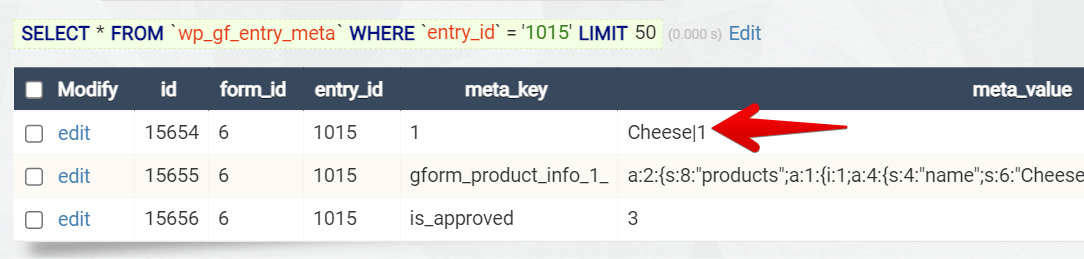# How to target a specific field value with [gravitymath] filters

The "filter" parameter only works with `scope="view"` or `scope="form"`. More about that here: The [gravitymath] Shortcode.

Assuming you have a form like this one: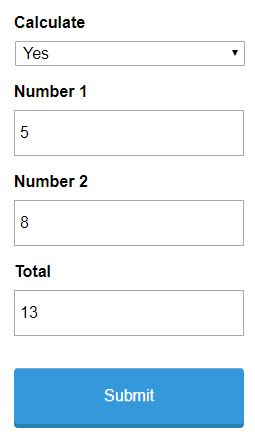...and a View like this one: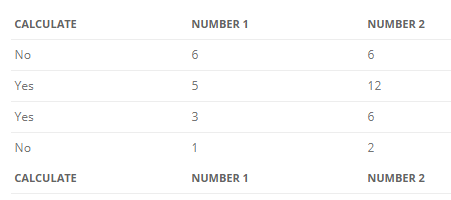Now, you want to calculate the sum between Number 1 and Number 2, only for the entries where the value of the Calculate field is "Yes". That's now possible with the "filter" parameter of the [gravitymath] shortcode.

Here's our shortcode:

```Total: [gravitymath scope="view" id="8" filter="filter_1=Yes&op_1=is"]{Number 1:2}+{Number 2:3}[/gravitymath]
```

...and here's the output in the View: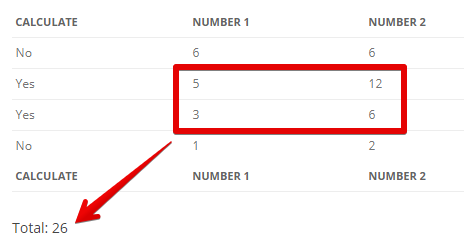### Usage

The following attributes for the "filter" parameter are supported:

`filter_N` - the filter, where N is the ID of the field or meta key
`op_N` - the operation for a corresponding `filter_N`, default: `is`, other operators are `isnot`, `contains`, `notcontains`, `lt` (less than), and `gt` (greather than).
`limit` - the number of entries to calculate. Leave it blank to use all entries from the chosen "scope" parameter.

In the example below, we are filtering the field Calculate (ID = 1) for the "Yes" value.

```[gravitymath scope="view" id="206" filter="filter_1=Yes&op_1=is"]...[/gravitymath]
```

If we wanted to filter by two fields instead, for example, Calculate =Yes and Number 2 = 6, then we'll do it like this:

```[gravitymath scope="view" id="206" filter="filter_1=Yes&op_1=is&filter_3=Yes&op_3=is"]...[/gravitymath]
```

Pay attention to the fact that filter_1 and op_1 correspond to the first field Calculate (ID = 1), and filter_3 and op_3 correspond to the field Number 2 (where ID = 3).

### Use values on the filter parameter, not labels

If you are filtering a choice-based field, like a dropdown, that uses values instead of only labels, then the filter will look for the value and not the label: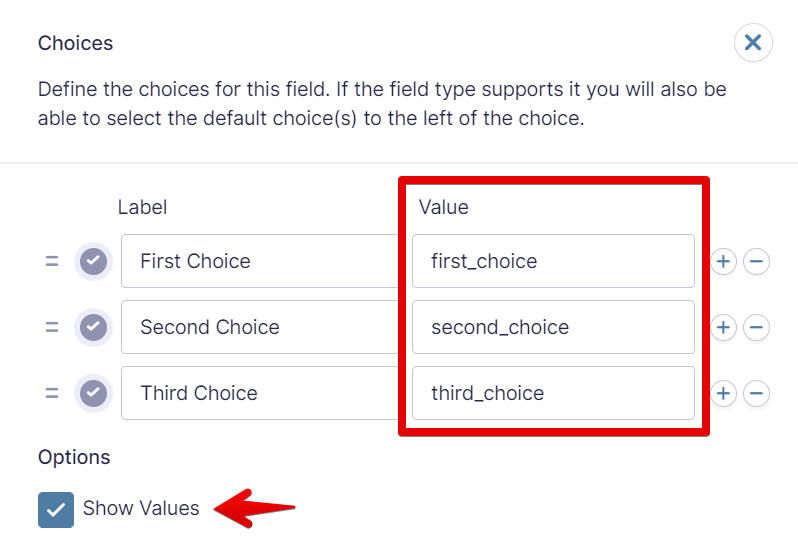Example:

```[gravitymath ... filter="filter_1=first_choice"]...[/gravitymath]
```

### When filtering product field choices, use the "contains" operator

Product field choices are stored in the database as `value|price`; therefore, when trying to filter for specific choices, use `contains` as the filter operator.

```[gravitymath scope="view" id="206" filter="filter_1=Cheese&op_1=contains"]...[/gravitymath]
```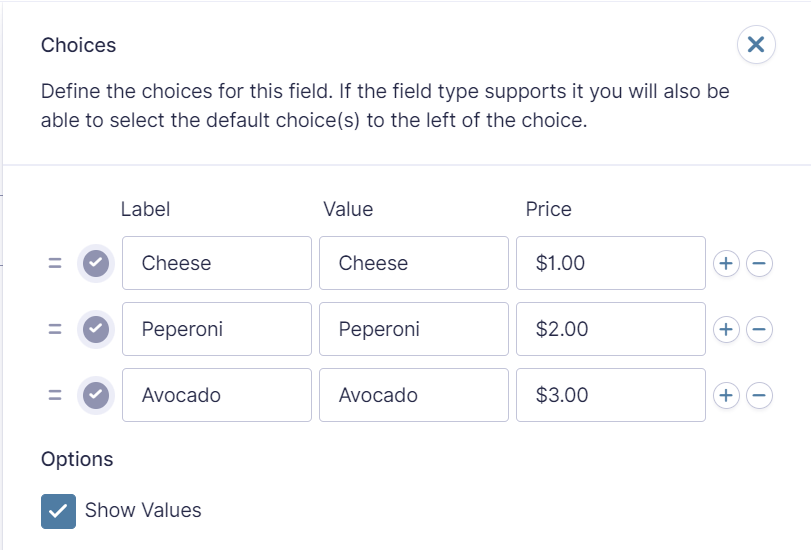How the choice is stored in the database: